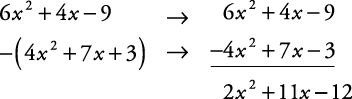Polynomials are expressions containing one or more than one term, with each term separated from the preceding one by a plus or minus sign. The exponents on the variables in a polynomial are always whole numbers. A polynomial has no maximum length. Some arithmetic operations with polynomials need just common sense, but others require special techniques.

In order to add and subtract polynomials successfully, you must understand what monomials, binomials, and trinomials are; what constitutes “like terms”; and the difference between ascending and descending order.

## Monomial, Binomial, and Trinomial

A monomial is an expression that could be a numeral, a variable, or the product of numerals and variables. If the expression has variables, certain restrictions apply to make it a monomial.

• Variables must have whole number exponents.

• Variables do not appear under simplified radical expressions.

• Denominators do not contain variables.

The following expressions are examples of monomials.

–12, a, 3 t 2,, y 3,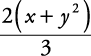The following are expressions that are not monomials.A binomial is an expression that is the sum of two monomials.

A trinomial is an expression that is the sum of three monomials.

A polynomial is an expression that is a monomial or the sum of two or more monomials.

## Like Terms or Similar Terms

Two or more monomials with identical variable expressions are called like terms or similar terms. The following are like terms, since their variable expressions are all x 2 y:

5 x 2 y, –3 x 2 y,The following are not like terms, since their variable expressions are not all the same:

–5 x 2 y 2, 4 x 2 y,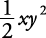In order to add monomials, they must be like terms. Unlike terms cannot be added together. To add like terms, follow this procedure.

2. Keep the variable expression.

3. ##### Example 1

Find the following sums.

1. 4 x 2 y + 8 x 2 y

2. –9 abc + 3 abc

3. 9 xy + 7 x – 28 xy – 4 x

1. 12 x 2 y

2. –6 abc

3. –19 xy + 3 x

Note that in answer (c), because –19 xy and 3 x are unlike terms, they cannot be added together.

## Ascending and Descending Order

When working with polynomials that involve only one variable, the general practice is to write them so that the exponents on the variable decrease from left to right. The polynomial is then said to be written in descending order.

When a polynomial in one variable is written so that the exponents increase from left to right, it is referred to as being written in ascending order.

##### Example 2

Rewrite the following polynomial in descending powers of x.

4 y 4 + 12 – 15 x 2 + 13 x 3 y + 17 xy 2

13 x 3 y – 15 x 2 + 17 xy 2 + 4 y 4 + 12

To add two or more polynomials, add like terms and arrange the answer in descending (or ascending if asked) powers of one variable.

##### Example 3

Find the following sum:>

• ( x 2 + x 3 – 3 x) + (4 – 5 x 2 + 3 x 3) + (10 – 8 x 2 – 5 x)

• ( x 3 + 3 x 3) + ( x 2 – 5 x 2 – 8 x 2) + (–3 x – 5 x) + (4 + 10)

• = 4 x 3 – 12 x 2 – 8 x + 14

This problem can also be added vertically. First rewrite each polynomial in descending order, one above the other, placing like terms in the same column.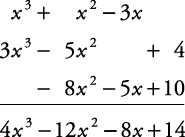To subtract one polynomial from another, add its opposite.

##### Example 4

Subtract (4 x 2 – 7 x + 3) from (6 x 2 + 4 x – 9).

Done horizontally,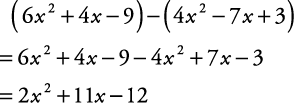Done vertically,